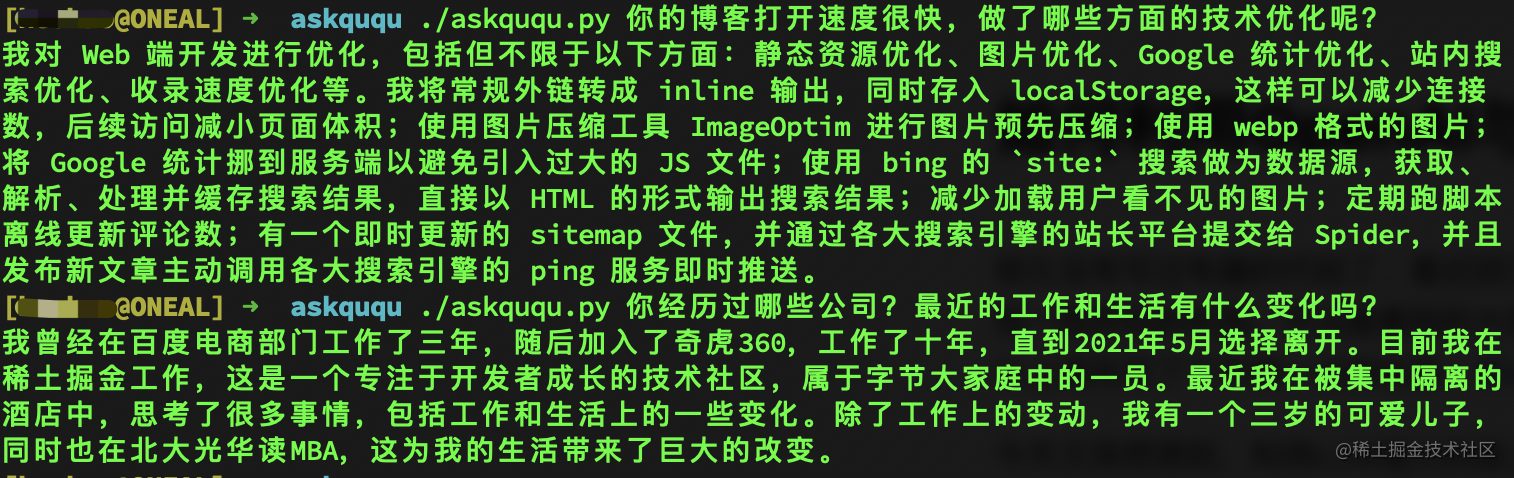```bash

# 注：文章摘要 by ChatGPT, 頭圖 by DALL·E

```## 實現原理

TL;DR: 先將文章內容通過 OpenAI 的 Embedding 模型轉化成向量數據，然後與問題進行向量距離計算，最後將topK答案扔給 OpenAI 潤色輸出。

### 第二步，優化內容token長度

```python import os import tiktoken

tokenizer = tiktoken.get_encoding("cl100k_base") posts = []

# 假如我們第一步先將文章內容保存到了本地./posts 目錄下

for file in os.listdir('posts/'): with open('posts/' + file, 'r', encoding='UTF-8') as f: text = f.read() [title, content] = text.split('@@@') tokens = len(tokenizer.encode(content)) posts.append({'title': title, 'content': content, 'tokens': tokens}) ``` tiktoken 是 OpenAI 提供的一個工具，可以快速標記文本成模型 token 以計算其長度。

```python def split_text(text, max_tokens = max_tokens): sentences = text.split('。') #按句號分割，以防將同一個句子分割到了不同的text斷中 n_tokens = [len(tokenizer.encode(" " + sentence)) for sentence in sentences] chunks = [] tokens_so_far = 0 chunk = []

``````for sentence, token in zip(sentences, n_tokens):
if tokens_so_far + token > max_tokens:
chunks.append("。".join(chunk) + "。")
chunk = []
tokens_so_far = 0
if token > max_tokens:
continue

chunk.append(sentence)
tokens_so_far += token + 1
return chunks
``````

ebd_posts = [] for post in posts: if post['content'] is None: continue if post['tokens'] > max_tokens: splited_posts = split_text(post['content']) for _post in splited_posts: ebd_posts.append((post['title'], _post)) else: ebd_posts.append((post['title'], post['content'])) ```

### 第三步，打標

```python import pandas as pd import openai

df = pd.DataFrame(ebd_posts, columns = ['title', 'content']) df['tokens'] = df.content.apply(lambda x: len(tokenizer.encode(x)))

# 以下這行代碼會多次請求 OpenAI 接口，會有較長的等待時間，如果數據量大可以自行做優化處理

df['embeddings'] = df.content.apply(lambda x: openai.Embedding.create(input=x, engine='text-embedding-ada-002')['data']['embedding'])

df.to_csv('data/embeddings.csv') df.head() ``` 同時，我們將打標後的數據保存在本地，方便後續查詢。因為我們這裏只是一個 demo 演示，所以使用本地文件存儲，如果內容量比較大的，建議使用向量數據庫。

### 第四步，計算匹配內容

```python import pandas as pd import numpy as np import openai from openai.embeddings_utils import distances_from_embeddings

max_len = 4000 #4096 for gpt-3.5-turbo

def match_text(question): context = [] cur_len = 0

``````# 問題打標

# 使用 OpenAI 提供的工具函數做向量匹配，如果是存儲的向量數據庫，查詢計算更方便
df['distances'] = distances_from_embeddings(q_embeddings, df['embeddings'].values, distance_metric='cosine')
for i, row in df.sort_values('distances', ascending=True).iterrows():
cur_len += row['tokens']
if cur_len > max_len:
break
context.append(row['content'])
return context
``````

``` 補充一句，因為 OpenAI 的 Embedding 模型是基於普遍性數據訓練，如果你的內容或問答過於專業，有可能就會出現查詢數據不準確的情況，這種時候可以考慮自己訓練 Embedding 模型，比如可參考 text2vec 這個項目。

### 第五步，優化回答內容

``````try:
# prompt 可以自己調整優化，其他參數也可以根據需要修改
completion = openai.ChatCompletion.create(
model='gpt-3.5-turbo',
messages=[
{'role': 'system', 'content': f"你叫xxx，是一個熱心和大家分享和交流的人，你擅長的領域有xxxx。"},
{'role': 'user', 'content': f"根據context提供的信息回答我提出的問題。\n\nContext: {context}\n\n---\n\nQuestion: {question}\nAnswer:"}
]
)
return completion['choices']['message']['content']
except Exception as e:
print(e)
return ''
``````

```

「其他文章」